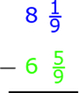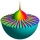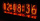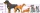# Sum of mixed

The sum of two mixed numbers is 15½. One of the number is 5 ¾, what is the other?

x =  9.75

### Step-by-step explanation:Did you find an error or inaccuracy? Feel free to write us. Thank you!

Showing 1 comment:Math Student
Q: What is 14 1/4 plus 6 7/8

A: 21 1/8 . See details of calculation: https://www.hackmath.net/en/calculator/fraction?input=14%201/4%20plus%206%207/8## Related math problems and questions:

• Simplify 3Simplify mixed numerals expression: 8 1/4- 3 2/5 - (2 1/3 - 1/4) Show your solution.
• Sum of fractionsWhat is the sum of 2/3+3/5?
• Mixed numbersRewrite mixed numbers, so the fractions have the same denominator: 5 1/5 - 2 2/3
• Linear combination of complexIf z1=5+3i and z2=4-2i, write the following in the form a+bi a) 4z1+6z2 b) z1*z2
• Fraction + eqSolve following simple equation with fractions: -5/6(8+5b) = 75 + 5/3b
• Turtles 2A box turtle hibernates in the sand at 11 5/8. A spotted turtle hibernates at 11 16/25 feet. Which turtle is deeper? Write answer as number 1 or 2.
• ComplaintsThe table is given: days complaints 0-4 2 5-9 4 10-14 8 15-19 6 20-24 4 25-29 3 30-34 3 1.1 What percentage of complaints were resolved within 2weeks? 1.2 calculate the mean number of days to resolve these complaints. 1.3 calculate the modal number of day
• Manufacturing companyA random sample of 8 manufacturing companies is selected from a population of manufacturing companies. The market values (in millions of rands) of these eight manufacturing companies are: 17 65 117 206 172 181 221 94 What is the lowest and highest market
• EmilyEmily had 20 minutes to do a three-problem quiz. She spent 11 3/4 minutes on question A and 5 1/2 minutes on question B. How much time did she have left for question C?
• Fractions and mixed numerals(a) Convert the following mixed numbers to improper fractions. i. 3 5/8 ii. 7 7/6 (b) Convert the following improper fraction to a mixed number. i. 13/4 ii. 78/5 (c) Simplify these fractions to their lowest terms. i. 36/42 ii. 27/45 2. evaluate the follow
• The sum 12The sum of 3 mixed numbers is 20 13/15. two of the numbers are 6 1/3 and 7 5/6. what is the third number?
• Watching TVOne evening 2/3 of students watch TV. Of those students, 3/8 watched a reality show. Of the students that watched the show, 1/4 of them recorded it. What fraction of the students watched and recorded reality tv.
• Statistical XY fileYear; money spent on advertising; profit (three values each row) 2008 2 12 2009 5 20 2010 7 25 2011 11 26 2012 15 40 1. draw a scatter diagram depicting the data. 2. calculate the Pearson's correlation coefficient. 3. determine the linear regression equat
• Evaluate 5Evaluate expression x2−7x+12x−4 when x=−1
• LCD two fractionsWhat is the least common denominator of 11/15 and 12/19?
• Eq2 2Solve following equation with quadratic members and rational function: (x2+1)/(x-4) + (x2-1)/(x+3) = 23
• BoatsThree-quarters of boats are white, 1/7 are blue and 9 are red. How many boats do we have?